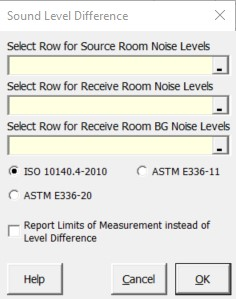### Strutt Help

Sound Level Difference    1/1, 1/3

Strutt|Building Acoustics|Sound Level Difference calculates the sound level difference between a source and receiver room, taking into account the background noise level of the receiver room:#### ISO 10140-4 (2010)

Where the background noise level in the receiver room is 15 dB or more below the measured receiver room noise level:
L=L_(source)-L_(receiver)

Where the background noise level in the receiver room is 6 - 15 dB below the measured receiver room noise level:
L=L_(source)-10*log10(10^(L_(recevier)/10)-10^(L_(BGN)/10))

Where the background noise level in the receiver room is 6 dB or less below the measured receiver room noise level, it shall be clearly indicated in the report that a 1.3 dB correction has been made and these values are the limit of measurement:
L=L_(source)-1.3

If the user ticks the "Report Limits of Measurement instead of Level Difference" box, the function will report whether or not a correction to the receiver room level as been applied as follows:
• BGN 15 dB or more below receiver room level: "Valid"
• BGN 6-15 dB below receiver room level: "Correction"
• BGN 6 dB or less below receiver room level: "Limit"

#### ASTM E336-11

Where the background noise level in the receiver room is more than 10 dB below the measured receiver room noise level:
L=L_(source)-L_(receiver)

Where the background noise level in the receiver room is 5 - 10 dB below the measured receiver room noise level:
L=L_(source)-10*log10(10^(L_(recevier)/10)-10^(L_(BGN)/10))

Where the background noise level in the receiver room is less than 5 dB below the measured receiver room noise level, it shall be clearly indicated in the report that a 2 dB correction has been made and these values are the limit of measurement:
L=L_(source)-2

If the user ticks the "Report Limits of Measurement instead of Level Difference" box, the function will report whether or not a correction to the receiver room level as been applied as follows:
• BGN More than 10 dB below receiver room level: "Valid"
• BGN 5-10 dB below receiver room level: "Correction"
• BGN less than 5 dB below receiver room level: "Limit"

#### ASTM E336-20

Where the background noise level in the receiver room is 6 dB or more below the measured receiver room noise level:
L=L_(source)-10*log10(10^(L_(recevier)/10)-10^(L_(BGN)/10))

Where the background noise level in the receiver room is less than 6 dB below the measured receiver room noise level, it shall be clearly indicated in the report that a 1.26 dB correction has been made and these values are the limit of measurement:
L=L_(source)-1.26

If the user ticks the "Report Limits of Measurement instead of Level Difference" box, the function will report whether or not a correction to the receiver room level as been applied as follows:
• BGN 6 dB or more below receiver room level: "Valid"
• BGN less than 6 dB below receiver room level: "Limit"